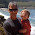## Wednesday, February 9, 2011

### Get a Random Value Within a Given Range

Just the code:

`    public int getRandomValue(long min, long max) {        return (int) Math.max(min, (int) (Math.random() * max) + 1);    }`

Use this to (obviously) generate an integer value between (inclusively) the given minimum and maximum - which is useful also to fetch random elements e.g. from a List.

1.Hi,

your proposed code will give you the min value more often depending on the entered values.
Example: getRandomValue(95, 100) will statistically give you the value 95 in 95 % of the cases (when (Math.random() * max) + 1 is lower than 96.

Better is something like this:

return (int) (Math.random() * (max - min)) + min;

Cheers

2.Nice catch, Thorben - thanks!